### Home > CALC > Chapter 3 > Lesson 3.2.1 > Problem3-51

3-51.
1. Evaluate each limit. If the limit does not exist, say so but also state if y is approaching positive or negative infinity. Homework Help ✎

1.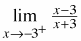2.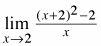3.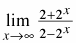4.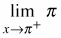(Careful!)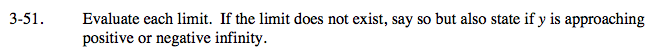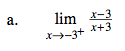The denominator does not cancel out. That means that there will be a 0 in the denominator when we evaluate at x = −3, so the limit does not exist.

Graphically, there will be a vertical asymptote at x = −3. That is why we are asked to find the limit from the right: we want to know if the graph approaches the asymptote towards +∞ or −∞.

So evaluate a value that is to the right of −3, and see if you get a positive or negative answer.

$\lim_{x\rightarrow -2.9}\frac{(-)}{(+)}=-\text{value}$

Therefore, from the right, the graph of

$y=\frac{x-3}{x+3}$

approaches the vertical asymptote towards −∞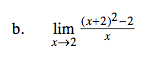Evaluate.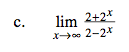This is a limit →∞. Think end behavior. Compare the highest power on the top and bottom. Be sure to consider their coefficients.

$\lim_{x\rightarrow \infty }\frac{2+2^{x}}{2-2^{x}}=\lim_{x\rightarrow \infty }\frac{2^{x}}{-2^{x}}=-1$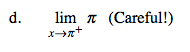Think: The function is y = π. That is a horizontal line. All y-values are π.